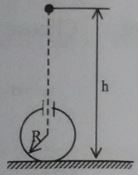# How many will succeed?Electrically charged drops of mercury fall from an altitude $h$ into a spherical metal vessel of radius $R$ in the upper part of which there is a small opening. The mass of each drop is $m$ and the charge of the drop is $q$. The number $n$ of the last drop that can still enter the sphere is $\large n*2\pi \epsilon_0 \frac{mg(h-R)R}{q^2}$. Then what is the value of $n$?

×